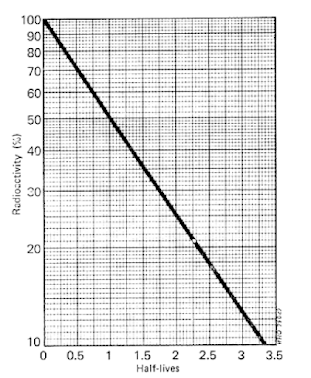Half-life of Radiopharmaceutical Preparations : Pharmaguideline -->
 Editable Pharmaceutical Documents in MS-Word Format

Radiopharmaceutical preparations are characterized by their shelf-life (expiration date) by the physical half-life of the radioisotopes.

## Half life

Radiopharmaceutical preparations are characterized by their shelf-life (expiration date) by the physical half-life of the radioisotopes, their radiochemical stability, and the radio-nuclidic impurities they contain. Radiopharmaceutical preparations usually contain radioisotopes with very short half-lives, so the shelf-life of such preparations is very limited. Expiration dates and times are so indicated with these preparations.

Radioactivity decreases from its original value to one-half after a certain amount of time has passed. Atoms decay at the same rate for each radionuclide and at their own characteristic rate. It follows that exponential decay curves are described mathematically as follows:

N = N0λ −2t

A radionuclide's characteristic disintegration constant is the number of atoms at a given temperature, and a radionuclide's number of atoms N0 is its atomic number at a given time.. As a function of disintegration constant, half-lives are related by the equation:

N1/2 = 0.693/ λ

For radioactive decay corrections, one can either use the exponential equation or decay tables, or obtain a decay curve for the given radionuclide associated with the correction.### Physical half life

Radiation decays one-half of its original value within the physical half-life of a radionuclide (T1/2 p). Even though the decay time of an individual atom cannot be determined, the decay rate of a large number of atoms can be calculated based on statistical considerations. It is constant and characteristic for each individual radionuclide to decay at a constant rate for a collection of atoms (N). Here's what is known about exponential decay:

N = N0 e −µt

The number of nuclei at time t equals N, while N0 represents the number of nuclei at zero time, and denotes the decay constant. A decay law equation is commonly used to describe this relationshipBy using the decay law relationship, one can determine the activity of a quantity of radioactive substance at any other point in time if the activity of the quantity is known at one time. Half-life and decay constant are generally interconnected in the following way:

T1/2 = 0.693/µ

Along with the use of decay law formulas, radioactivity can also be measured by plotting decay curves for the specified radionuclide or using decay tables.

### Biological half life

Radiopharmaceutical half-life (T1/2b) can be defined as the period between when the concentration in a tissue, organ, or entire body drops 50% of its maximum level, without taking into account radioactive decay.

### Effective half life

The half-life of a radiopharmaceutical (T1/2e) is determined by how the radioactive substance interacts with its physical and biological half-lives within tissues, organs, or all the body. In order to determine what dose of radiopharmaceutical to administer and to monitor the level of radiation exposure, the effective half-life is essential. Here is how it is calculated:

T1/2e = T1/2p × T1/2b/T1/2p × T1/2b

Physical half-lives T1/2p and biological half-lives T1/2b are the respective half-lives.
Get subject wise printable pdf documents

Ankur Choudhary is India's first professional pharmaceutical blogger, author and founder of pharmaguideline.com, a widely-read pharmaceutical blog since 2008. Sign-up for the free email updates for your daily dose of pharmaceutical tips.

## CURRENT JOBS

 Show All ❭❭Jobs by PharmaJobs

## DOCUMENTS

PHARMACEUTICAL DOCUMENTS

Editable Pharmaceutical Documents in MS-Word Format. Ready to use SOPs, Protocols, Master Plans, Manuals and more...

View